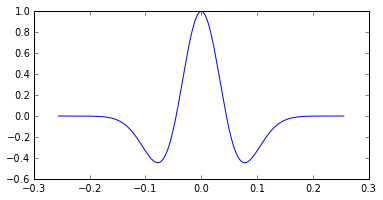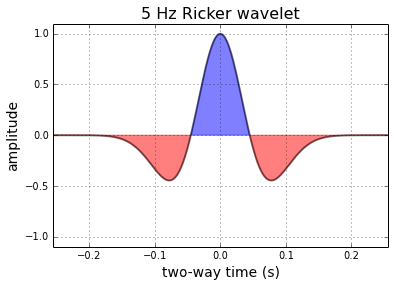This is a IPython notebook tutorial for defining a ricker wavelet function, and plotting it. The iPython notebook is designed to be used seamlessly with Matplotlib. When you start your own notebook, type: ipython notebook --pylab inline

In :
%pylab inline

Populating the interactive namespace from numpy and matplotlib

In :
import numpy as np
import matplotlib.pyplot as plt

def ricker(f, length=0.512, dt=0.001):
t = np.linspace(-length/2, (length-dt)/2, length/dt)
y = (1.-2.*(np.pi**2)*(f**2)*(t**2))*np.exp(-(np.pi**2)*(f**2)*(t**2))
return t, y


Here we define the function "ricker" and state that it needs 3 input paramaters; frequency, f, the length of time over which we want it to be defined, length, and the sample rate of the signal, dt. Calling the function returns two arrays, the time axis (t), and the value of the function, y.

To create a 5 Hz ricker wavelet, assign the value of 5 to the variable f, and pass it into the function like so,

In :
f = 5
t, y = ricker (f)


To plot the result:

In :
plt.plot(t, y)
savefig( '5Hz_ricker_basic.png', facecolor='white')But with a few more commands, we can improve the cosmetics,

In :
plt.figure(figsize=(6,4))
plt.plot( t, y, lw=2, color='black', alpha=0.5)
plt.fill_between(t, y, 0,  y > 0.0, interpolate=False, hold=True, color='blue', alpha = 0.5)
plt.fill_between(t, y, 0, y < 0.0, interpolate=False, hold=True, color='red', alpha = 0.5)

# Axes configuration and settings (optional)
plt.title('%d Hz Ricker wavelet' %f, fontsize = 16 )
plt.xlabel( 'two-way time (s)', fontsize = 14)
plt.ylabel('amplitude', fontsize = 14)
plt.ylim((-1.1,1.1))
plt.xlim((min(t),max(t)))
plt.grid()
savefig( '5Hz_ricker_pretty.png', facecolor='white')In [ ]: